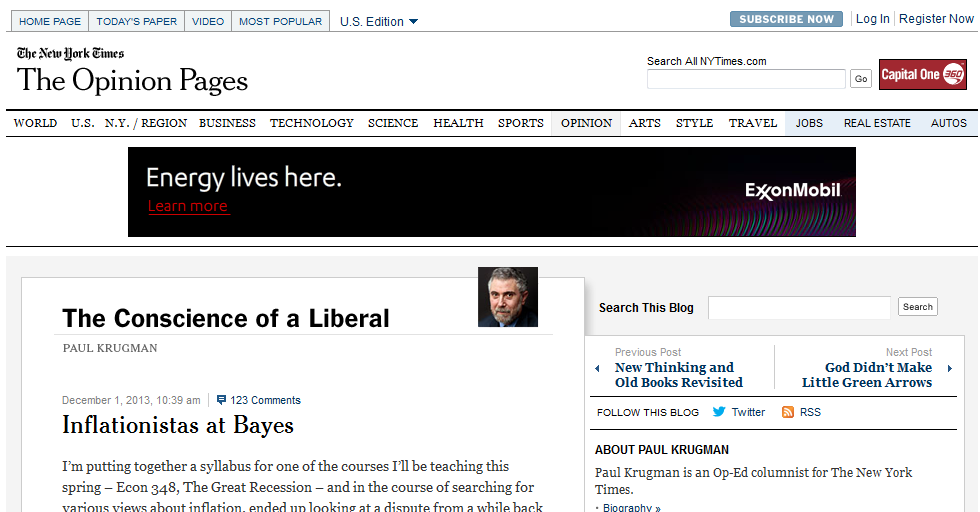# PMean: The cost of a bad prediction

Paul Krugman wrote up an interesting application of Bayes Theorem on his blog on the New York Times. I want to adapt his example and expand it a bit.

Suppose that two experts are making a prediction about inflation. The first expert predicts that inflation is likely in the upcoming year because of [insert your favorite Economic theory here]. The second expert predicts that inflation is unlikely in the upcoming year because of [insert a competing Economic theory here]. The year comes and goes and there is no inflation. The second expert criticizes the first expert and says “Your prediction was wrong, so you have no credibility.” The first expert replies, “I only said that inflation was likely. I didn’t say it would happen with absolute certainty. So my prediction was not wrong a probabilistic sense.”

So, what do you think? Should a probabilistic forecast be considered “wrong” if it doesn’t occur? If not, is there any penalty for the credibility of someone who makes a forecast of a “likely” event that does not occur.

Dr. Krugman suggests Bayes theorem as a way to evaluate this setting. I’m changing his numbers slightly, but it does not change the general concept that he was trying to illustrate. Suppose that “likely” means a probability of 80% and “unlikely” means a probability of 10%. Place an uniformative prior probability on each expert of 50%. This means that the probability that the first expert makes more accurate predictions than the second expert is equal to the probability that the second expert makes more accurate predictions than the first expert.

Mathematically, this can be shown as follows.

E1=first expert makes more accurate predictions than the second expert.

E2=second expert makes more accurate predictions than the first expert.

I=inflation in the coming year.

P[E1] = P[E2] = 0.5

P[I | E1] = 0.8; P[I | E2] = 0.1

Now apply Bayes Theorem to get P[E1 | not I]

P[E1 | not I] = P[not I | E1] * P[E1] / P[not I]

where P[not I] = P[not I | E1] * P[E1] + P[not I | E2] * P[E2]

The numerator is (1-0.8)*0.5 and the denominator is (1-0.8)*0.5+(1-0.1)*0.5. This makes

P[E1 | not I] = 0.182.

So when inflation does not occur, the credibility of the first expert drops from a prior probability of 0.5 to a posterior probability of 0.182. That means that one bad prediction does not mean that all your future predictions will be bad, but it does mean that you should trust the first expert a bit less.

Now this calculation depends a lot on what you assign for the probabilities of “likely” and “unlikely”. If the first experts quantification of “likely”  meant 0.6 and not 0.8, then you get

P[E1 | not I] = 0.333.

The first expert takes a hit, but not as serious. On the other hand, if “likely” really meant 0.95, then you get

P[E1 | not I] = 0.053.

Now this bit of mathematics is a gross oversimplification of how you should evaluate expert predictions, but it illustrates that you should avoid assigning very high probabilities to certain events, unless you are really really sure of yourself. Otherwise, if your prediction is wrong, you take a big hit to your credibility.

Here’s a link to the original entry in Dr. Krugman’s blog.